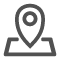# 解决类别不平衡数据方法介绍

## 1、类别不平衡数据集基本介绍

pip3 install imbalanced-learn

from sklearn.datasets import make_classification
import matplotlib.pyplot as plt

#使用make_classification生成样本数据
X, y = make_classification(n_samples=5000
n_features=2,  # 特征个数= n_informative（） + n_redundant + n_repeated
n_informative=2,  # 多信息特征的个数
n_redundant=0,   # 冗余信息，informative特征的随机线性组合
n_repeated=0,  # 重复信息，随机提取n_informative和n_redundant 特征
n_classes=3,  # 分类类别
n_clusters_per_class=1,  # 某一个类别是由几个cluster构成的
weights=[0.010.050.94],  # 列表类型，权重比
random_state=0)

#查看各个标签的样本量
from collections import Counter
Counter(y)

# Counter({2: 4674, 1: 262, 0: 64})

# 数据集可视化
plt.scatter(X[:, 0], X[:, 1], c=y)
plt.show()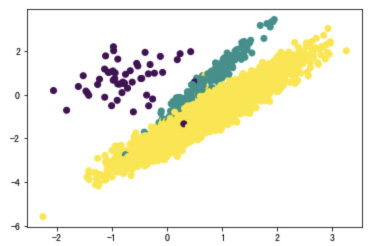## 2.1.1 什么是过采样方法

2.1.2 随机过采样方法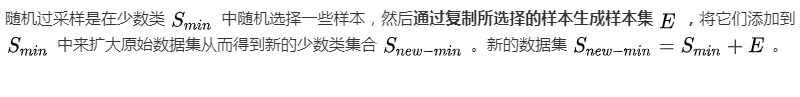# 使用imblearn进行随机过采样
from imblearn.over_sampling import RandomOverSampler
ros = RandomOverSampler(random_state=0)
X_resampled, y_resampled = ros.fit_resample(X, y)
#查看结果
Counter(y_resampled)
#过采样后样本结果
# Counter({2: 4674, 1: 4674, 0: 4674})

# 数据集可视化
plt.scatter(X_resampled[:, 0], X_resampled[:, 1], c=y_resampled)
plt.show()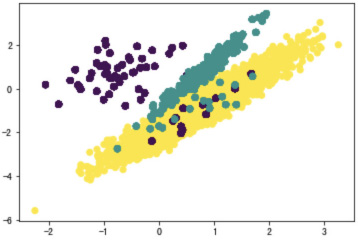·对于随机过采样，由于需要对少数类样本进行复制来扩大数据集，造成模型训练复杂度加大。

·另一方面也容易造成模型的过拟合问题，因为随机过采样是简单的对初始样本进行复制采样，这就使得学习器学得的规则过于具体化，不利于学习器的泛化性能，造成过拟合问题。

## 2.1.3 过采样代表性算法-SMOTE

SMOTE全称是Synthetic Minority Oversampling即合成少数类过采样技术。

SMOTE算法是对随机过采样方法的一个改进算法，由于随机过采样方法是直接对少数类进行重采用，会使训练集中有很多重复的样本，容易造成产生的模型过拟合问题。而SMOTE算法的基本思想: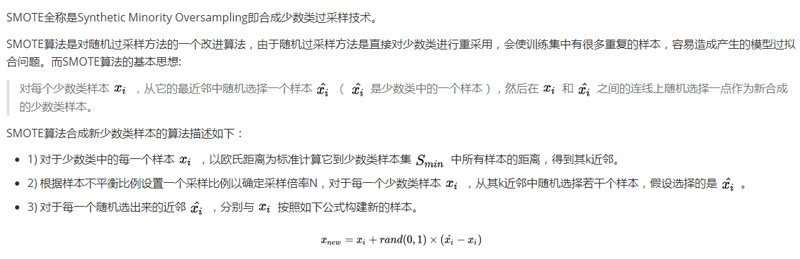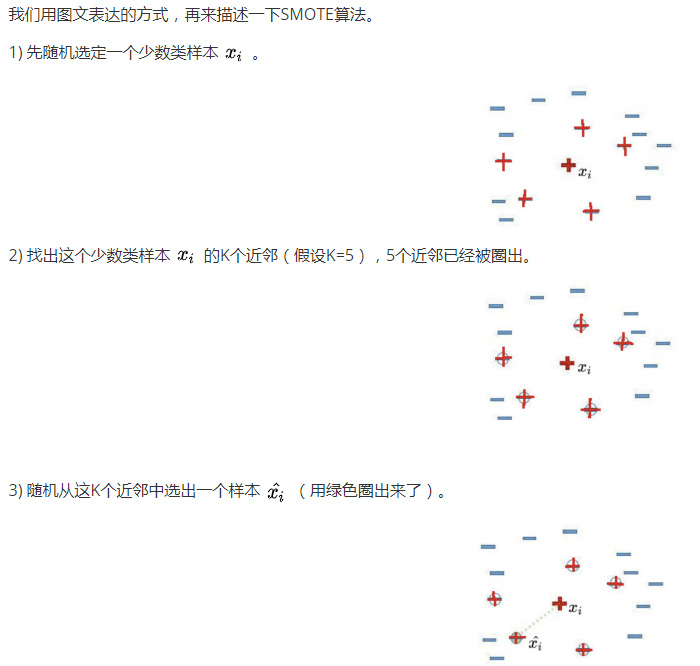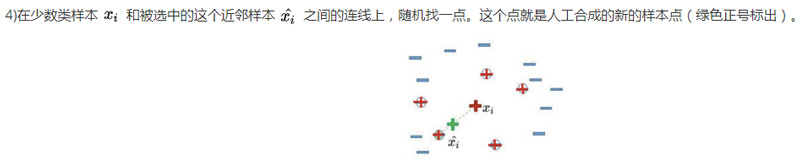# SMOTE过采样
from imblearn.over_sampling import SMOTE
X_resampled, y_resampled = SMOTE().fit_resample(X, y)
Counter(y_resampled)

# 采样后样本结果
# [(0, 4674), (1, 4674), (2, 4674)]

# 数据集可视化
plt.scatter(X_resampled[:, 0], X_resampled[:, 1], c=y_resampled)
plt.show()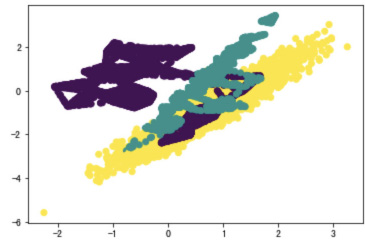## 2.2.1 什么是欠采样方法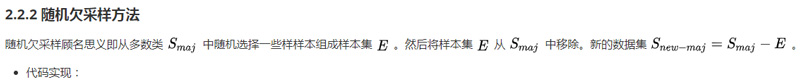# 随机欠采样
from imblearn.under_sampling import RandomUnderSampler
rus = RandomUnderSampler(random_state=0)
X_resampled, y_resampled = rus.fit_resample(X, y)
Counter(y_resampled)

# 采样后结果
[(064), (164), (264)]

# 数据集可视化
plt.scatter(X_resampled[:, 0], X_resampled[:, 1], c=y_resampled)
plt.show()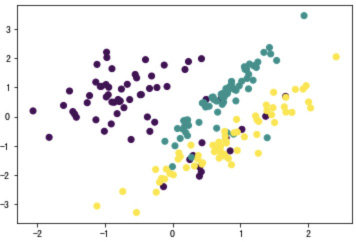python人工智能之人脸识别综合应用与实践